Dichronauts

Double Plus, Double Minus: A Gentle Introduction to the Physics of Dichronauts

The four-dimensional universe we inhabit has three dimensions of space and one of time. But what would it be like to live in a universe where the roles were divided up more evenly, so that there were two of each: two dimensions of space, and two of time?

It might be tempting to think that with two dimensions of time, the history of any particle, or person, would cease to be linear and would take on some kind of planar aspect. But in fact, there is no reason why objects shouldn’t trace out essentially one-dimensional world lines through space-time, much as they do in our own universe. The difference that arises from having a second dimension of time is that of all the straight lines that pass through a given point, more of them would count as possible world lines.

Once we choose a particular world line as our personal history, the three-dimensional space perpendicular to it will obey a strange kind of geometry, in which the roles played by circles and spheres in our universe are taken by hyperbolas and hyperboloids, light can only travel in certain directions, and some rivers will flow uphill. These are just some of the strange phenomena to be found in the world of Dichronauts.

World Lines in the Dichronauts Space-TimeA world line is the line traced out over time by an object, where the object is idealised as occupying a single point in space. But rather than just showing the object’s position in space at each moment, each point on a world line has four coordinates, including the time at which the object reaches the position described by the other three coordinates. Although this is tricky to visualise in all four dimensions, if we ignore one dimension of space we can draw world lines perfectly well in the remaining three dimensions, as in the image on the right.

In space-time diagrams like this, time is usually drawn as the vertical axis. So, an object that is standing still has a vertical straight line as its world line, while an object moving at a constant velocity will have a straight line that tilts away from the vertical, so that as we move forward in time by moving upwards along the line, the position of the object changes at a constant rate.

When discussing the geometry of space-time, it’s common practice to choose units in which the speed of light is exactly 1; for example, we could measure time in years and distances in light years. When we apply this choice of units to our space-time diagrams, it means that anything moving at the speed of light will have a world line at forty-five degrees from the vertical. The light cone in the diagram on the right marks this limit. And since ordinary objects must travel at less than the speed of light, all possible world lines that pass through the origin of our chosen coordinate system must lie inside the light cone.

The requirement of being inside the light cone can be expressed in terms of the four coordinates (x, y, z, t) of any point on the world line. Since x2 + y2 + z2 is the square of the total distance in space from the origin (this is just the three-dimensional version of Pythagoras’s Theorem), for an object that is travelling at less than the speed of light (which is 1, in our choice of units), x2 + y2 + z2 must be less than the square of the time that has passed, t2. In mathematical notation:

x2 + y2 + z2 < t2

We can rearrange this slightly to give:

x2 + y2 + z2t2 < 0

In this inequality, every coordinate of space, x, y, z, has a plus sign, while the sole time coordinate t has a minus sign. So if we switch to a universe where we have two dimensions of space, x, y, and two dimensions of time, t, u, we would expect the equivalent expression to have two plus signs and two minus signs:

x2 + y2t2u2 < 0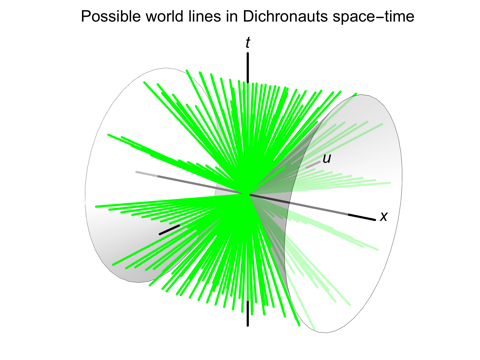If we drop one of the space coordinates, y, we can draw the possible world lines for the Dichronauts universe in three dimensions; they are shown in the image on the left. Here, rather than lying within a cone around the t-axis, the world lines are all excluded from a cone around the x-axis.

[This would give the complete picture in a universe with only one dimension of space, but we should keep in mind that we really do have a second dimension of space, y, that’s been omitted here. In four dimensions, there’s a perfect symmetry between the region in which world lines can lie and the region from which they are excluded.]

In any case, what we can see clearly in this diagram is that while the only possible world lines for objects moving in the x direction still need to lie at an angle of less than forty-five degrees from the t-axis, the world lines for objects that move in the u direction can make any angle at all with the t-axis. This means that in the u direction, there is no speed limit! And the same will hold true for any direction that is closer to u than to x.

So, even in a universe with two dimensions of time, we can talk sensibly about the history of an object as a linear sequence of events: a world line. The difference is, for objects moving at high speed, the usual speed-of-light limit has been removed for a range of directions, which opens up more possibilities for the world lines.

Geometry and Rotations in the Dichronauts Space

While the plethora of possible world lines are important when objects can have very high velocities, in our own everyday life most objects we encounter are travelling at very small fractions of the speed of light. This means that we, and all those objects, have world lines that are very nearly parallel. We can take the direction along our own world lines to be the time dimension of space-time, and other people — whose world lines are almost parallel to ours — will more or less agree with this choice.

Having settled on a specific direction for time, any direction perpendicular to it will be one that we consider to be purely a direction in space. In our own universe, that leaves us with three dimensions of space that all behave in the same way: there’s no fundamental difference between x, y and z.

But in the Dichronauts universe, if t is our chosen time dimension, we are left with three perpendicular dimensions, x, y and u, as “directions in space”, but they are not all fundamentally the same, because u is potentially a direction a world line could follow, while x and y are not. So although we have a three-dimensional space here, we can’t expect its geometry to be that of our own familiar, Euclidean space.

The recipe we followed before, when switching from the formula that tells us what world lines are possible in our universe to the one that works for the Dichronauts universe, was to change z2 to –u2. In Euclidean space, x2 + y2 + z2 is the square of the distance from the origin to the point (x, y, z). So in the three-dimensional space with two “space-like” dimensions, x and y, and one “time-like” dimension, u, we would expect x2 + y2u2 to tell us something about the distance from the origin to the point (x, y, u).

If x2 + y2u2 is positive, we can treat is as being exactly the square of the distance from the origin. But what if it is negative? We would then need to take the square root of the opposite expression, u2x2y2, as the distance.

This might seem strange, but what it is telling us is that there are two fundamentally different kinds of “distance” in this geometry: one where x2 + y2u2 is positive and one where it is negative. And this shouldn’t be too surprising, because the second kind is exactly what we get for a distance that lies along a potential world line. Although we’ve chosen coordinates so that t is the direction of our own world line, and we’re also assuming that most of the people and objects around us have world lines pointing in roughly the same direction, the mere fact that some of our “space” directions — directions perpendicular to t — are potentially the directions of world lines, and some are not, is enough to make the two kinds of direction qualitatively different.

Actually, there is a third kind of direction as well: those for which x2 + y2u2 is exactly zero. In Euclidean space, x2 + y2 + z2 can only be zero if x, y and z are all zero, which describes the single point at the origin, (0,0,0). But x2 + y2u2 will be zero across the surface of an entire cone.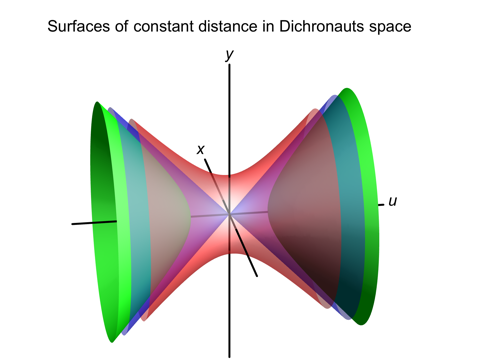In the image on the right, we can see surfaces of constant distance from the origin of all three types. The red, hourglass-like surface is for a positive value of x2 + y2u2. The two green, dish-like surfaces are for a negative value of x2 + y2u2. And the two blue cones that lie between them are for x2 + y2u2 = 0. (Because all three surfaces are translucent, the cones only look blue when there’s no other surface in front of or behind them).

The technical name for the red surface is a hyperboloid of one sheet, and the green surfaces comprise a hyperboloid of two sheets.

Having introduced a new way of measuring distance into our geometry, we might ask what it actually means, in a practical sense, to say that all the points on each of these surfaces lie at the same distance from the origin. When we talk about rotating an object — spinning a ball, swinging a stick, turning any solid object at all — we mean we are changing the positions of most of the points in the object, but we are not changing any of the distances between those points. In other words, the object remains rigid as we move it; we are not stretching or squashing it.

In Euclidean space, if we swung a stick around in such a way that one end of the stick remained fixed, the other end would always lie on a surface of constant distance from the fixed end — which in Euclidean geometry is a sphere. So, what these new surfaces of constant distance from the origin are showing us is where the end of a stick would have to lie, if we did the same thing in the Dichronauts space.

When we swing a stick in Dichronauts space, even though (by definition) its length remains constant, the distance it spans in other directions can grow arbitrarily large! For example, if we started with a stick of length 5 that pointed along the u-axis, we could swing it around so that its endpoint was at x=12, y=0, u=13, because in both case we would have x2 + y2u2 = –25. Similarly, if we started with a stick of length 5 that pointed along the x-axis, we could swing it around so its endpoint was at x=0, y=13, u=12, because in both case we would have x2 + y2u2 = 25. But although we can make these individual coordinates as huge as we like, the fixed relationship between them means that we could never swing the first kind of stick into any of the positions that are possible for the second kind of stick, and vice versa.If we rotate something in just two dimensions, the way it rotates will depend on whether we have two “space-like” dimensions, such as x and y, or one “space-like” dimension and one “time-like”, such as x and u. In the first case, the result will be exactly like rotating something in two-dimensional Euclidean space. In the second case, the lines of constant distance from the point around which we are rotating the object will be hyperbolas, rather than circles, and the object can be made to span arbitrarily large distances.

The image on the left shows examples of the two kinds of rotation. In both cases, a square is rotated back and forth around its centre, but in the second case, the farther it is rotated, the greater the distances it spans in the individual x and u directions. To our eyes, in the second case the square appears to be changing shape, as if it is being squashed along one diagonal and stretched along the other, but in the geometry of the Dichronauts space it is being rotated as a rigid object, with all the distances between its points remaining the same.

What’s more, since the square is being rotated as a rigid object, the angles between its sides cannot be changing either. So although it looks to us as if the corner angles are growing larger and smaller, the sides of the square are actually remaining perpendicular to each other all the time.

Light, and the Dark Cone

In our universe, if a pulse of light is emitted at time t=0 from the point x=0, y=0, z=0, then at some later time t we know that the distance it has travelled will be equal to the speed of light times t. If we make the usual choice of adopting units where the speed of light is 1, the distance travelled will be precisely equal to t. And if we square both quantities, they will still be equal. The square of the distance from the origin to some point (x, y, z) is x2 + y2 + z2, so we can write:

x2 + y2 + z2 = t2

or equivalently:

x2 + y2 + z2t2 = 0

When we ask for the corresponding equation in the Dichronauts universe, we follow the usual recipe of replacing z2 with –u2. So the coordinate along the world line of any pulse of light in the Dichronauts universe will satisfy the equation:

x2 + y2u2t2 = 0

Now, while this singles out a set of world lines in the full, four-dimensional space-time, we can also use it to discover something interesting about the possible paths that light can take in the three-dimensional Dichronauts space. If a pulse of light starts from the origin at time t=0, and ends up at some point in space (x, y, u), at a later time t, then if x2 + y2u2 were negative, it would be impossible for x2 + y2u2t2 to be zero, because you can’t subtract the positive number t2 from a negative number and end up with zero.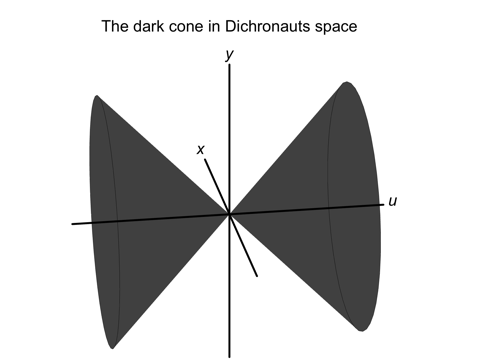It follows that there is a whole set of directions in the three-dimensional Dichronauts space, namely those for which:

x2 + y2u2 < 0

that can never be the direction of travel for a pulse of light!

These forbidden directions fill a cone around the u-axis that extends forty-five degrees away from the axis. This cone is not fixed to any one position in space; it describes a restriction on the possible directions for light that applies everywhere. So, no light source can emit a beam in any of these directions, and no one would be able to see anything by looking in a direction that lies within the dark cone.

We are used to thinking of any restriction so powerful that even light must obey it as applying to all material objects as well: for example, when we hear that light can’t escape from a black hole, it follows that no ordinary object, travelling even more slowly, could hope to escape either. But in the case of the dark cone, nothing could be further from the truth! As we saw earlier in the section on possible world lines, not only can ordinary objects move in the direction of the u-axis, they can do so at any speed at all.

So, the dark cone contains no light, but material objects are free to move in these directions. And since sound waves are nothing but vibrations in material objects, sound is also free to move in the dark cone.

Falling Uphill

Let’s suppose that the Dichronauts universe contains some kind of world, and that gravity attracts objects towards the surface of this world in the usual way. For now, we won’t go into the details of the overall shape such a world might take, and the way gravity would work on astronomical scales; these matters will be discussed elsewhere. We will just assume that we are looking at a small part of the surface of this world, where gravity is constant, and singles out a particular direction as “up”.

The direction “up” might be “space-like” or “time-like”; for example, it might be x, or it might be u, in our usual choice of coordinates. But whichever it is, the two directions in space that are perpendicular to “up” – the two that are horizontal – will have to include at least one direction that is of the opposite kind to “up”. What happens if we build a ramp that rises up as it stretches along that horizontal direction of the opposite kind, and then place an object on the ramp and see which way it slides?

This might sound like a stupid question, with the only possible answer being: down. We have already stipulated that gravity pulls things towards the surface in the usual way: if you drop something, it falls down in a straight line. But dropping something in mid-air is not the same as placing it on a ramp and letting it slide: the ramp exerts a force on the object, which adds to the force of gravity and modifies the object’s trajectory.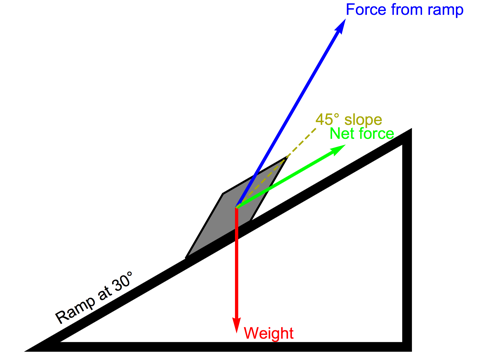The force exerted by the ramp will be perpendicular to the surface of the ramp. But as we have already seen with the odd appearance of the corners of a square when we rotate it in the x-u plane, lines that are perpendicular in this geometry do not look perpendicular in the Euclidean sense.

In the image on the left, we see an object sitting on a ramp that slopes at an angle of thirty degrees. The weight of the object (the red arrow) is pulling it down, while the force the ramp applies (the blue arrow), to keep the object from penetrating its surface, is perpendicular to the surface. The size of the force will be whatever is necessary in order for the net force to end up parallel to the surface of the ramp — but in this case, the final result (the green arrow) points upwards along the ramp.

To our eyes, the force from the ramp does not look perpendicular to the surface, but in the geometry of the Dichronauts space, it is. The characteristic of perpendicular lines in this geometry is that they both make the same angle with a line at forty-five degrees, and it’s not hard to see that the force from the ramp, and the net force (which is parallel to the surface) both make the same angle with the dashed line in the diagram.

So, unless there is enough friction to hold the object in place, the net force will make it slide up the ramp.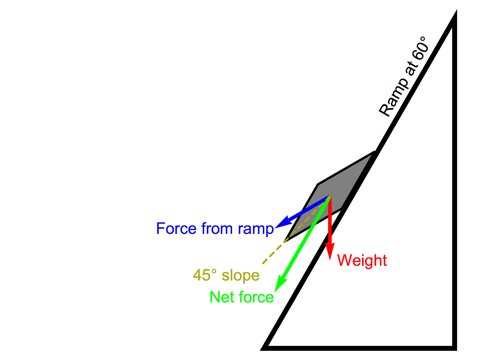However, if the ramp is steeper than forty-five degrees, the situation changes. The image on the right shows a sixty-degree ramp, and here the extra slope is enough to make the force from the ramp point downwards. The net force on the object will make it slide down, and in fact (neglecting the effects of friction) it will accelerate down the ramp even faster than it would fall straight down through the air.

The first case, with an object sliding up the ramp, might appear to violate the law of conservation of energy — but that is not the case once we take account of the necessary differences in the way energy is defined in the Dichronauts universe.

Since the force of gravity is directed vertically downwards, we will define gravitational potential energy to be a positive multiple of the height of the object above the ground. This is consistent with the kinetic energy associated with vertical motion always being positive. So far, this is the same as the situation on the surface of the Earth.

But because we have chosen the horizontal direction of the ramp to be of the opposite kind to the vertical direction, the kinetic energy associated with horizontal motion will have the opposite sign to that for vertical motion. If the ramp is at a slope of less than forty-five degrees, an object sliding along the ramp will be moving more rapidly in the horizontal direction than the vertical, so its overall kinetic energy will be negative. That enables it to ascend up the ramp, because its gain in gravitational potential energy is balanced by its negative kinetic energy.

Solid, Liquid, Cone Plasma

Negative kinetic energy opens up the possibility for a collection of objects to end up moving extremely rapidly, without any energy being fed into the system, if they all have velocities “near the cone”: that is, with components that are roughly equal in the space-like and time-like directions. As a consequence, in the Dichronauts universe, if an ideal gas (a collection of particles that only interact with each other when they come into contact) could exist at all, it would be thermodynamically unstable, with random collisions sending the particles’ velocities shooting off towards infinity.

But another consequence of the same geometry is that two particles whose coordinates, (x1, y1, u1) and (x2, y2, u2), are very different can nonetheless have a very small value for (x1x2)2 + (y1y2)2 – (u1u2)2. The strength of any interaction will always depend, at least in part, on that quantity. So the forces between particles that are widely separated, in the sense of their coordinates, but still near to each other’s cones will tend to bind the whole system together, and even at low densities the result will be more like a liquid than a gas.

So, we can expect three states of matter: solids, liquids, and, when the temperature is high enough or the density is low enough that the binding force no longer holds things in check, a very unstable state in which all the particles have velocities near the cone. We will call this last state a “cone plasma”.Dichronauts / Double Plus, Double Minus: A Gentle Introduction to the Physics of Dichronauts / created Sunday, 11 December 2016